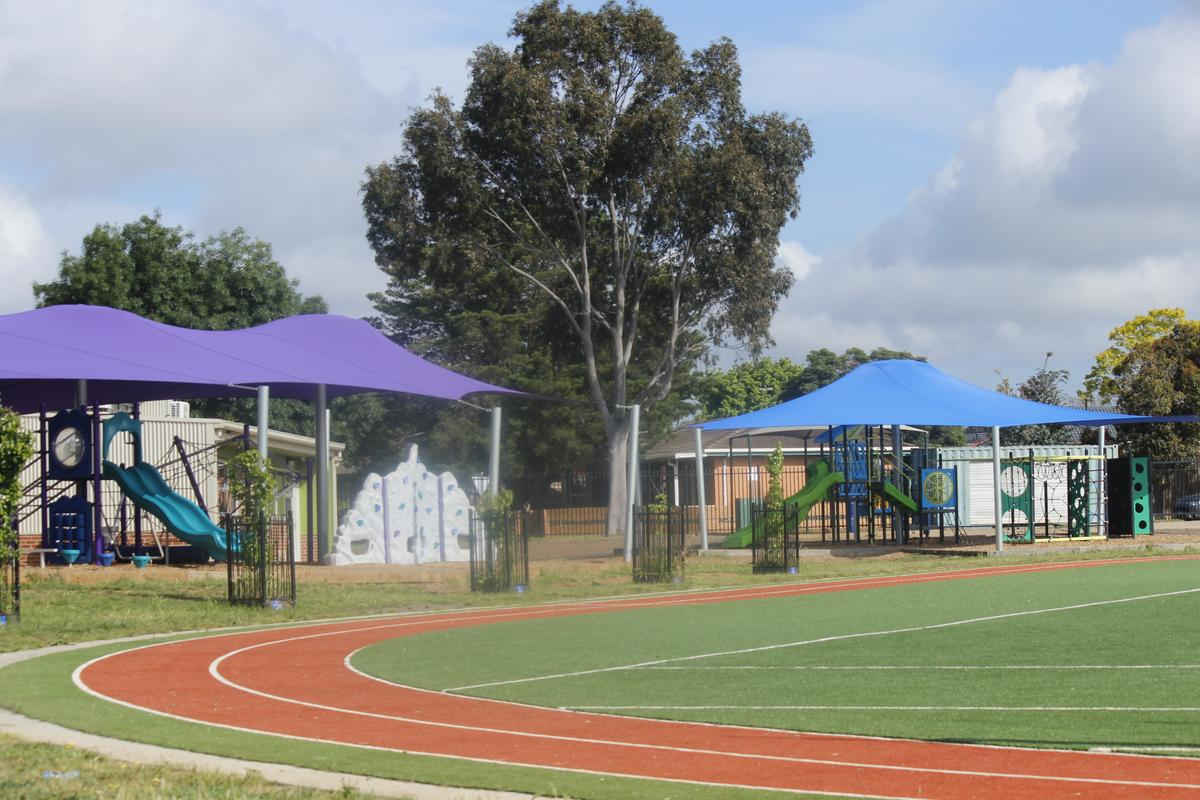# Numeracy

## WHAT DO WE LEARN IN MATHEMATICS?

Year One

Dear Parents and Carers,

Over the coming weeks I will continue to outline what the teaching and learning Mathematics curriculum encompasses for each year level. In this article I will focus on Year One. In the classroom children may be working at level, below level or above level. Teachers differentiate the teaching and tasks to cater for the learning each child needs to access.

In Level 1, students use mathematical symbols and language as well as materials and drawings in their mathematical explorations of daily life. There are 3three major areas Number and Algebra, Measurement and Geometry, Statistics and Probability.

Number and Algebra

Number and place value

• Develop confidence with number sequences to and from 100 by ones from any starting point. Skip count by twos, fives and tens starting from zero
• Recognise, model, read, write and order numbers to at least 100. Locate these numbers on a number line
• Count collections to 100 by partitioning numbers using place value
• Represent and solve simple addition and subtraction problems using a range of strategies including counting on, partitioning and rearranging parts
• Represent practical situations that model sharing.

Money and financial mathematics

• Recognise, describe and order Australian coins according to their value
• Recognise and describe one-half as one of two equal parts of a whole.

Patterns and algebra

• Investigate and describe number patterns formed by skip counting and patterns with objects
• Recognise the importance of repetition of a process in solving problems.

Measurement and Geometry

Using units of measurement

• Measure and compare the lengths, masses and capacities of pairs of objects using uniform informal units
• Tell time to the half-hour
• Describe duration using months, weeks, days and hours.

Shape

• Recognise and classify familiar two-dimensional shapes and three-dimensional objects using obvious features.

Location and transformation

• Give and follow directions to familiar locations.

Statistics and Probability

Chance

• Identify outcomes of familiar events involving chance and describe them using everyday language such as ‘will happen’, ‘won’t happen’ or ‘might happen.’

Data representation and interpretation